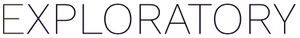# How to refer the number of rows in the Custom Function in Chart - n() function

#1

You can use `n()` R function to refer the number of rows in the Custom Function. For example, if you want to calculate the average of the profit by the sum of (Sales - Marketing) divided by the number of orders (number of rows), the definition of the Custom Function looks like the following.

``````sum(Sales - Marketing) / n()
``````

If you are new to the Custom Function, I suggest you to check How to Use Custom Function in Chart. It explains the concept of the Custom Function and some tips.